# Museum Blog

## Bedtime Buckaroos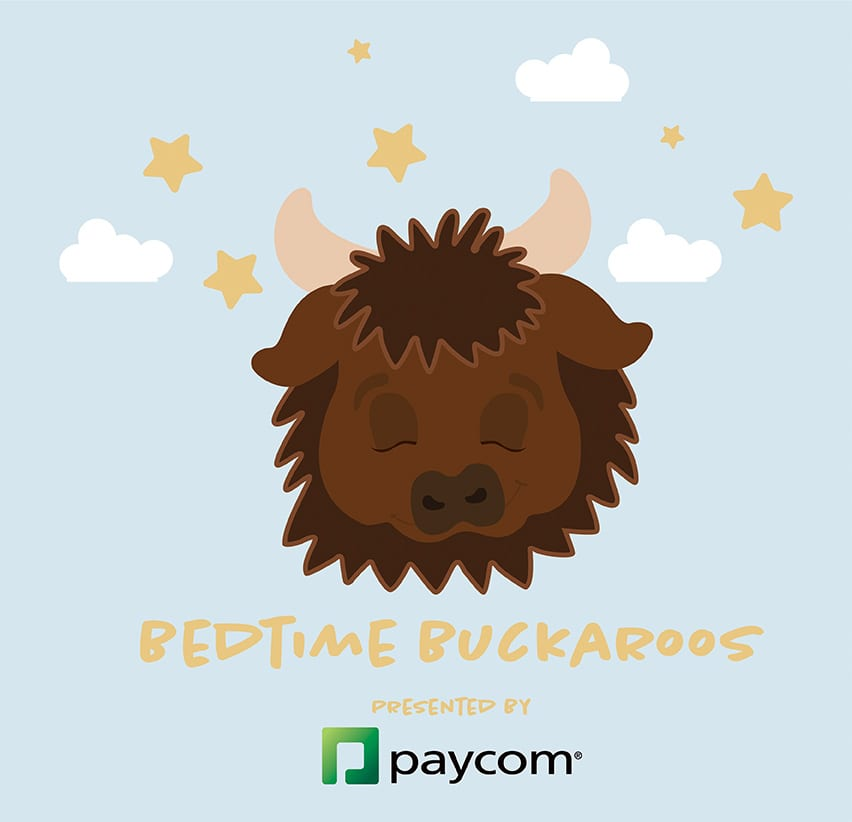Categorized In: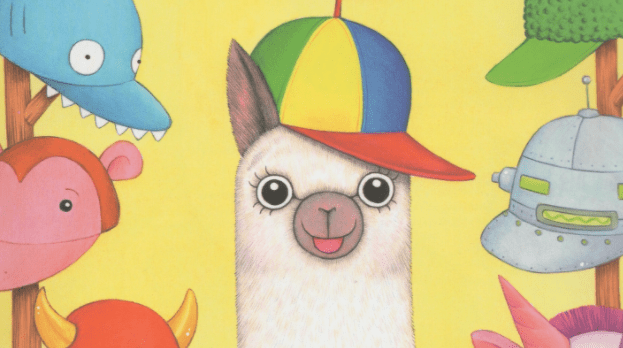Categorized In:Categorized In:Categorized In: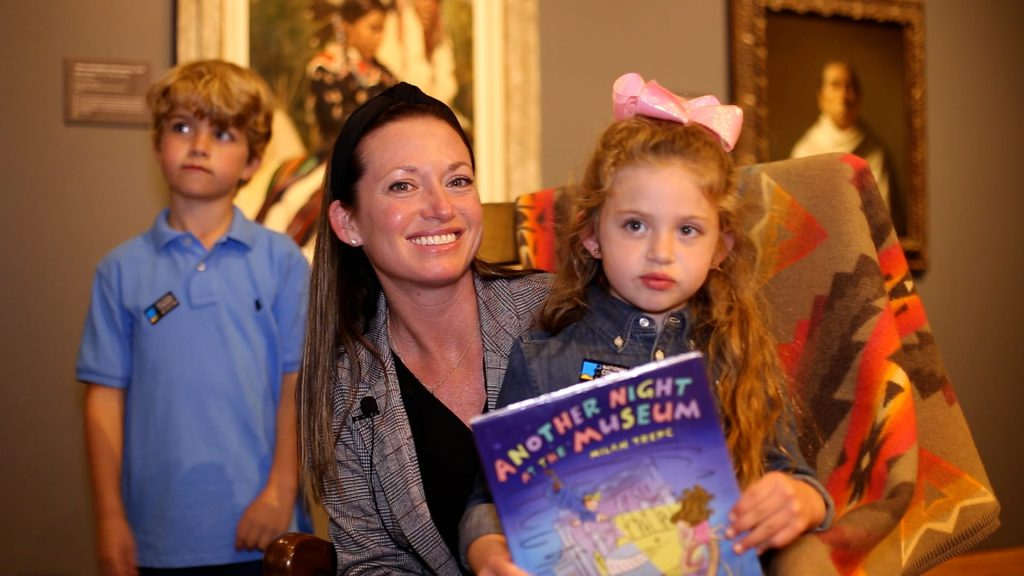Categorized In:Categorized In:Categorized In: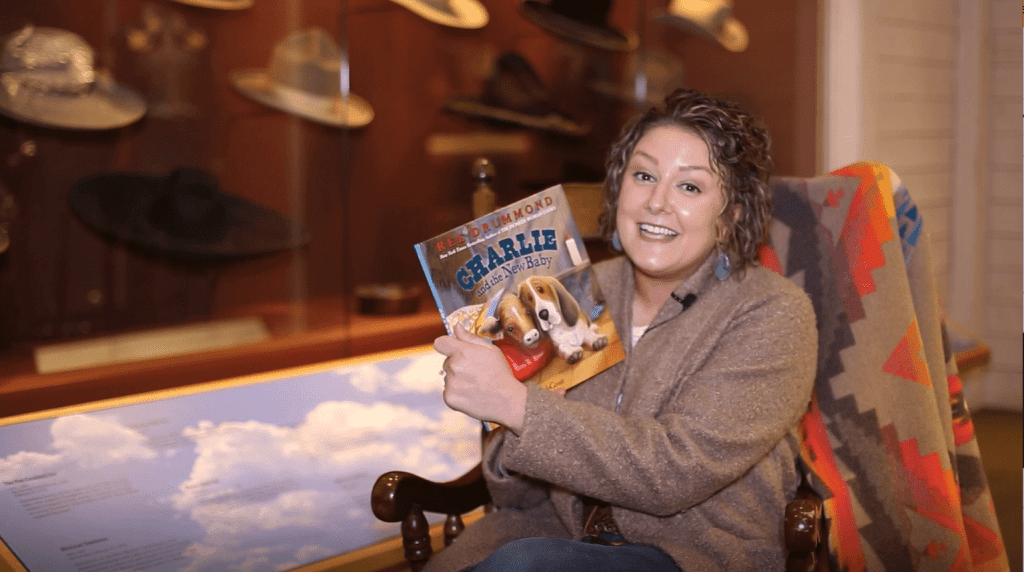Categorized In: ,Categorized In:Categorized In:Categorized In: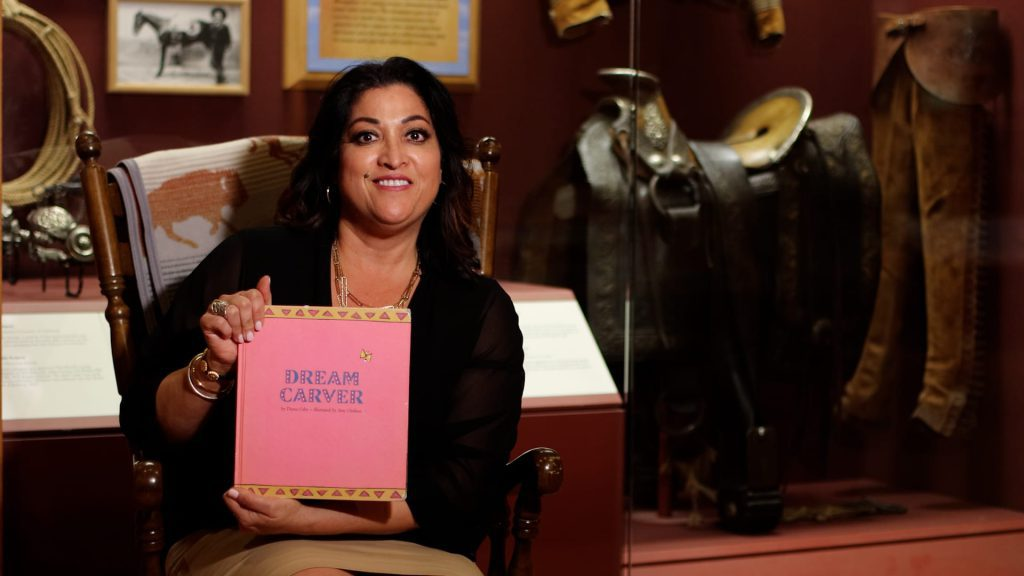Categorized In: ,Categorized In:Categorized In:Categorized In: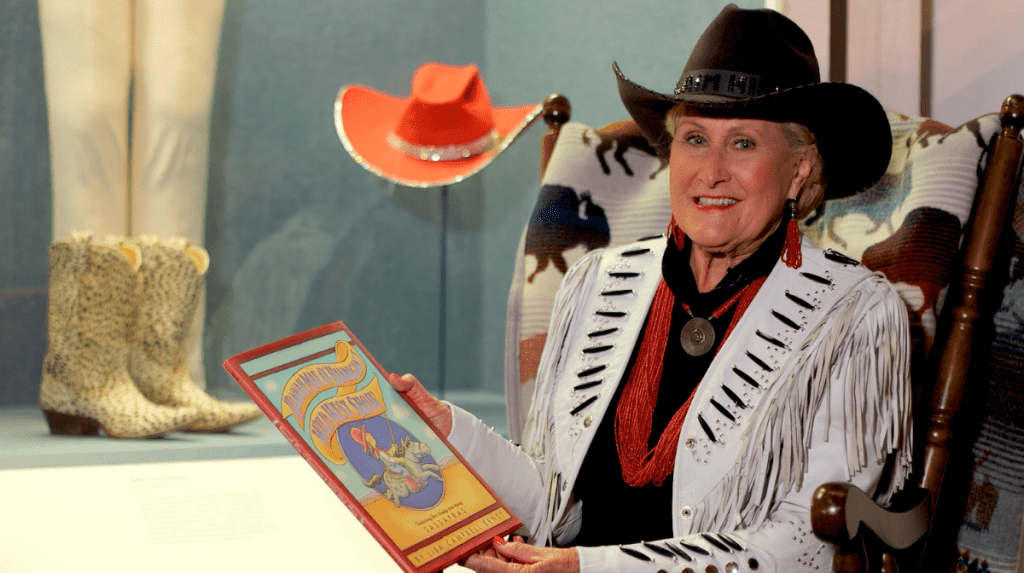Categorized In: ,Categorized In: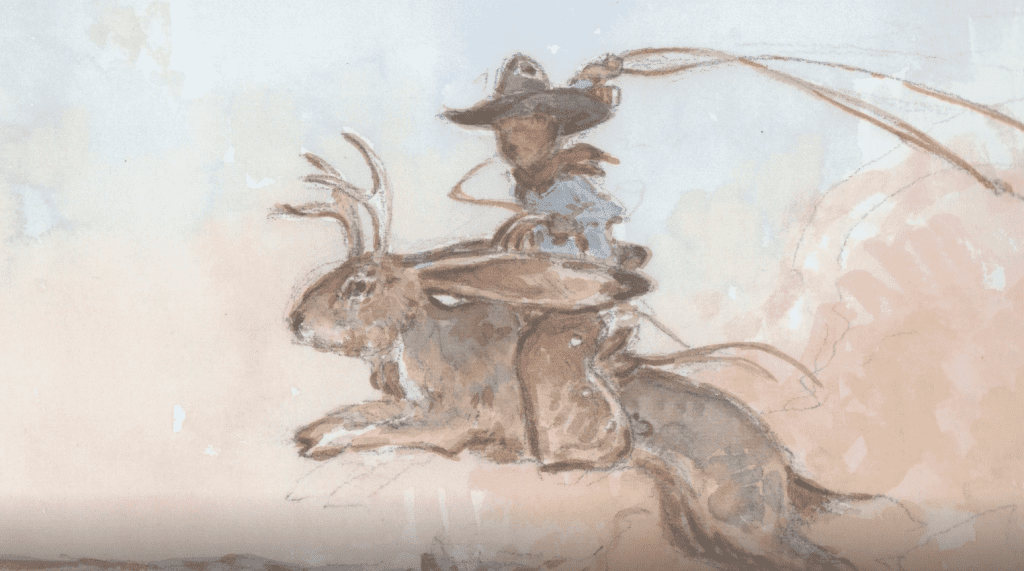Categorized In:Categorized In: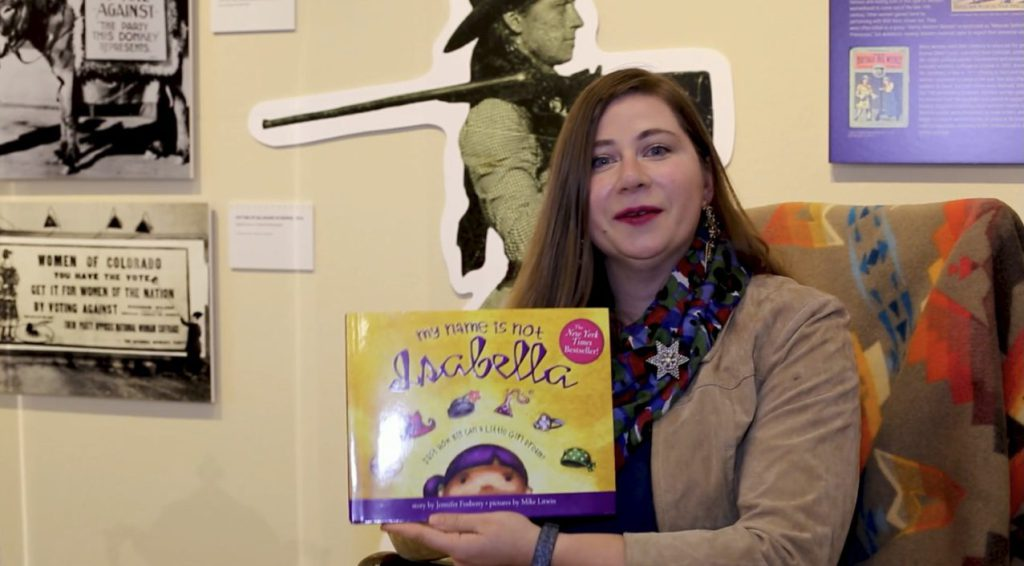Categorized In: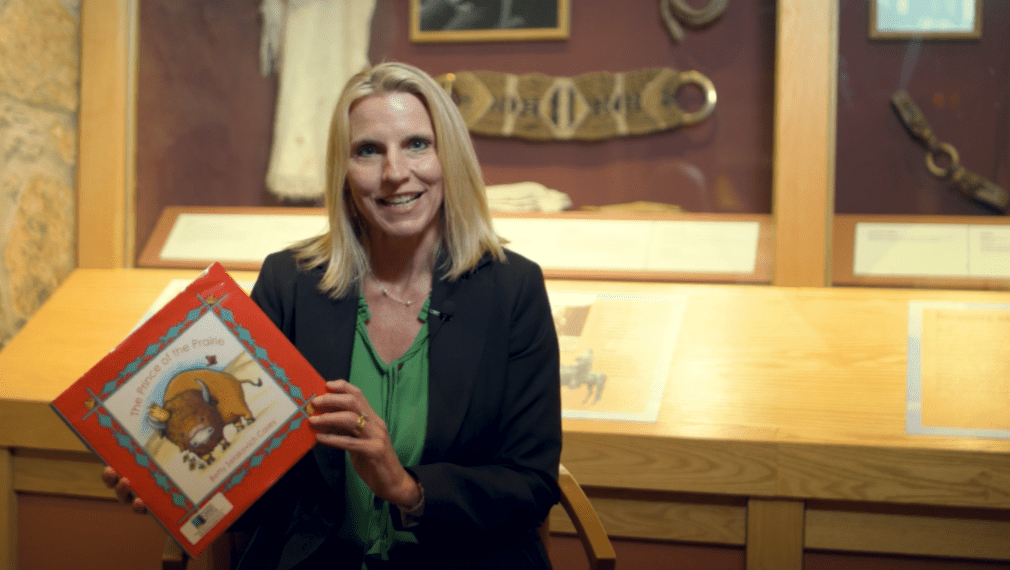Categorized In: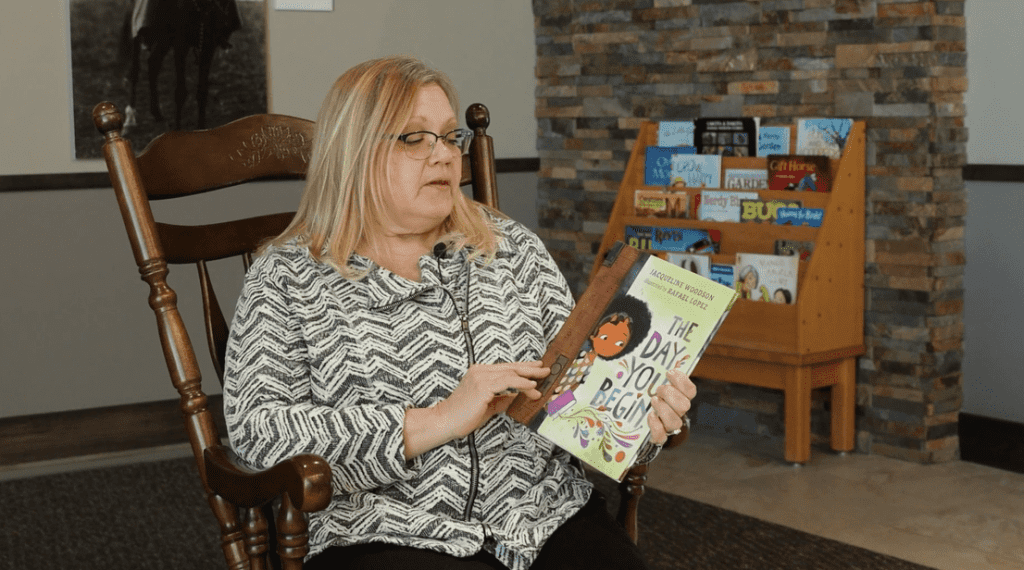Categorized In: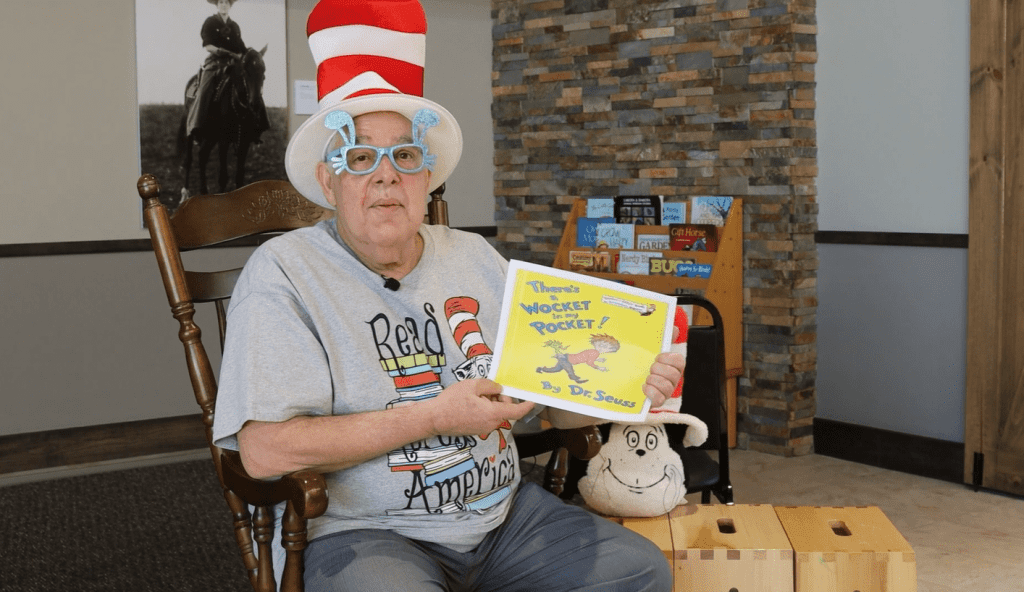Categorized In:Categorized In:Categorized In:Categorized In:Categorized In: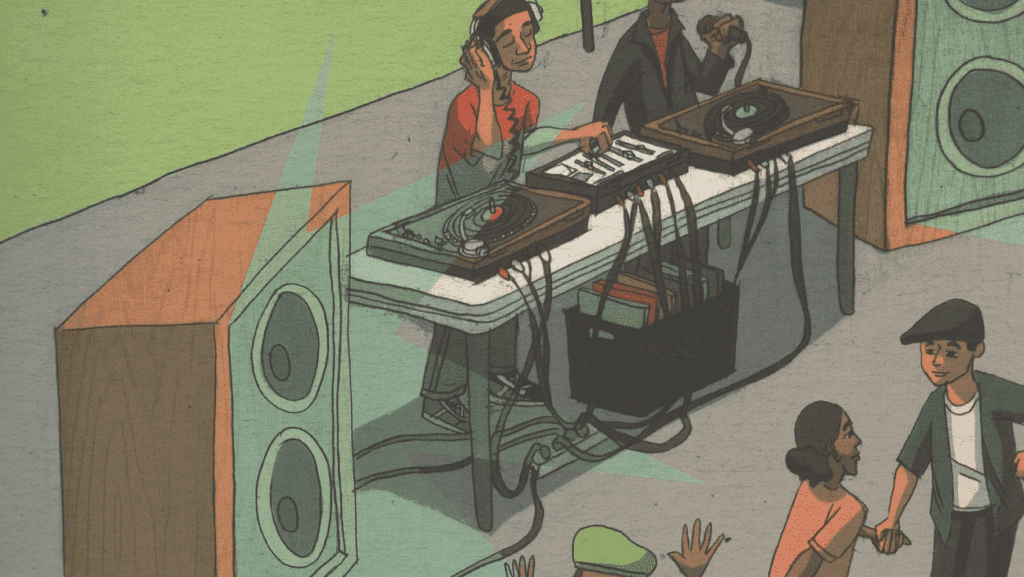Categorized In: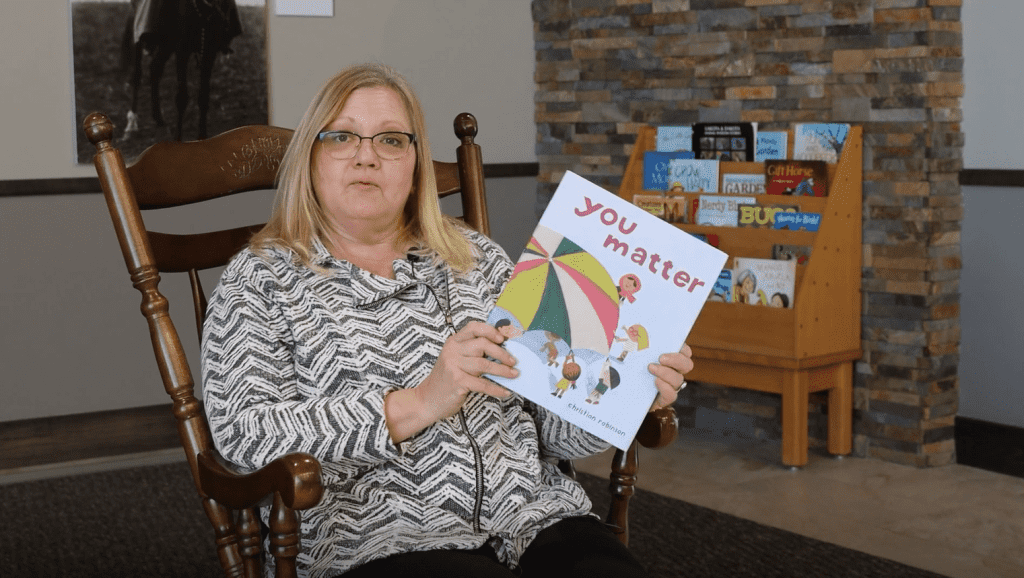Categorized In: ,Categorized In: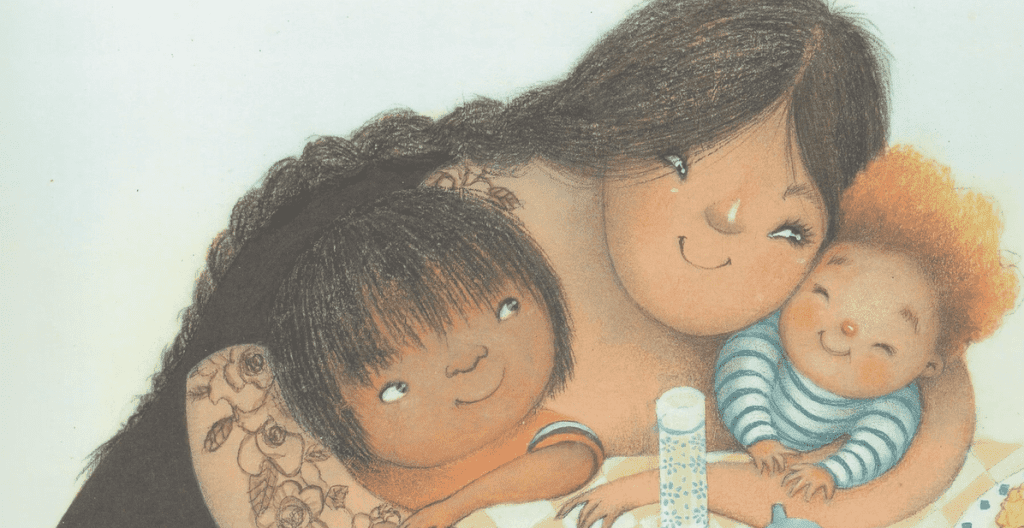Categorized In: ,Categorized In: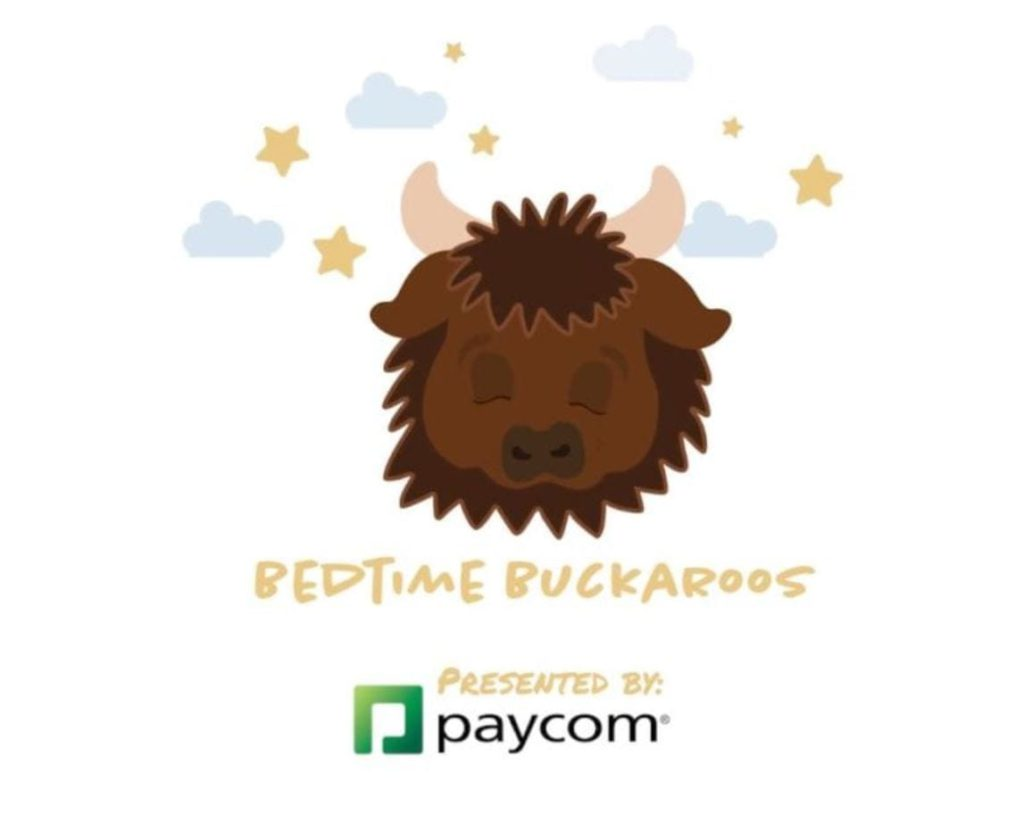Categorized In: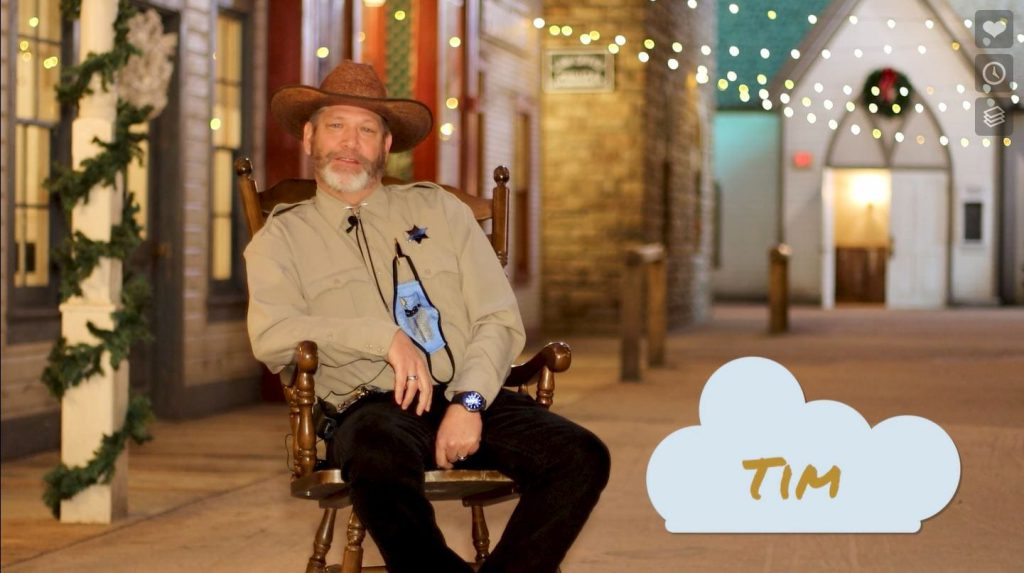Categorized In: